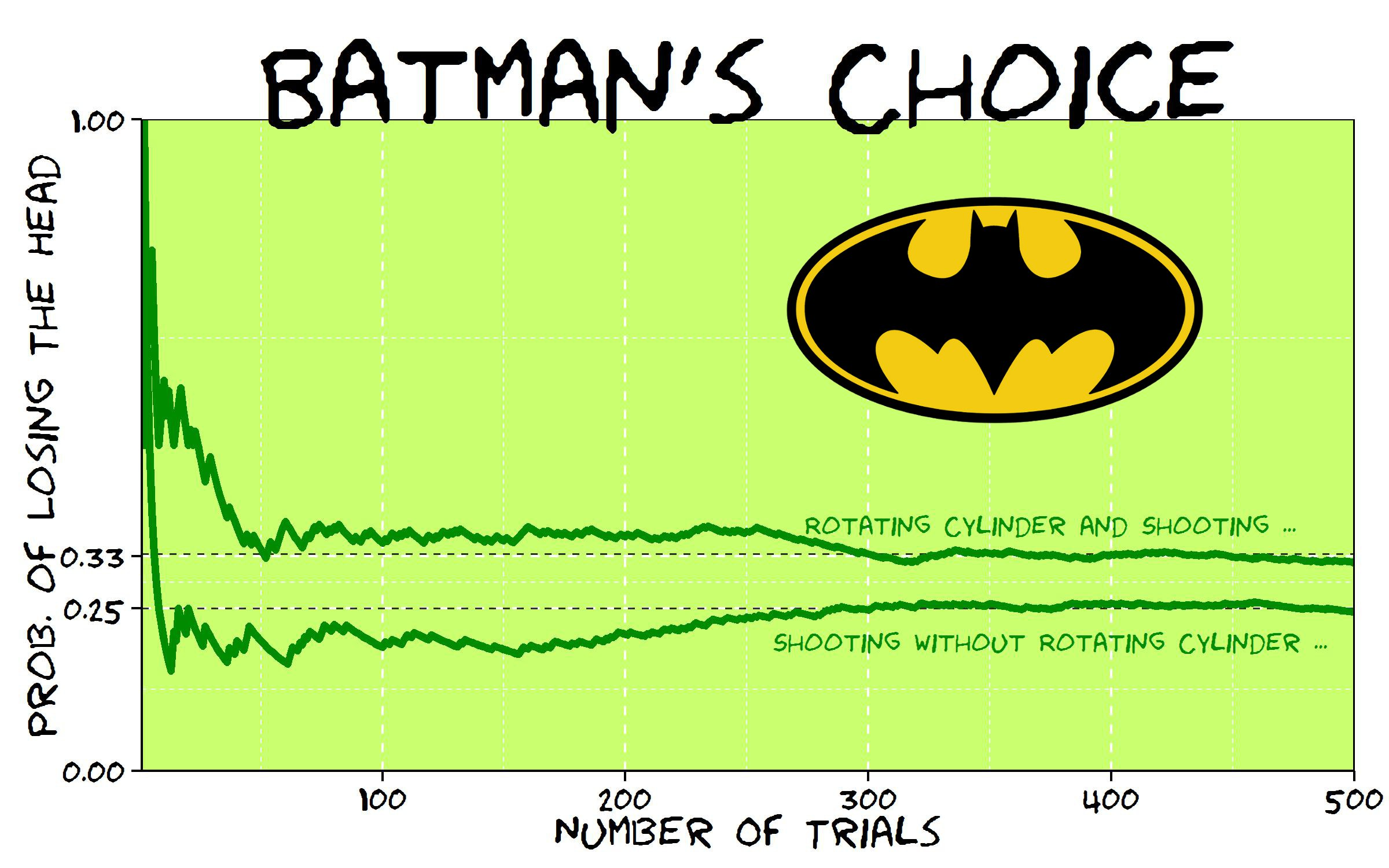# Batman’s Choice

A hero can be anyone, even a man doing something as simple and reassuring as putting a coat on a young boy’s shoulders to let him know the world hadn’t ended (Batman in The Dark Knight Rises)

Joker has captured Batman and keeps him into a dark and cold dungeon of Gotham City. Showing his sadistic character, Joker proposes the following game to Batman:

This is a six shooter revolver with two bullets in the cylinder. Bullets are inside two consecutive chambers. I will spin the cylinder and will fire the gun aiming to my head. If I survive you will have to do the same but you decide if you want to spin the cylinder before firing or not. If you still keep you head over your shoulders after firing, you will be free.

Joker fires and nothing happens. He survives and passes the revolver to Batman. What should Batman do? Spinning or not? What would you do?

From my point of view, answer is quite anti-intutive because the best option is not spinning the cylinder again. Spinning case is clear: probability of losing the head is 2/6=33% but what about not spinning? Doing the next shoot directly eliminates two possibilities: the previous shot of Joker and the second bullet according to direction of cylinder rotation (remember two bullets are consecutive and Joker is unfortunately  still alive). It means there is only 1 chance to dead between 4, so probability of losing the head in this scenario is 1/4=25% which is significantly lower than the first one.

Here you can find the resulting graph of simulating the game up to 500 times:Will it be the end of Batman? Not sure.

This is the code of this experiment:

```library(ggplot2)
library(extrafont)
niter=500
results=data.frame()
for (i in 1:niter)
{
bullet1=sample(1:6,1)
Joker=sample((1:6)[-c(bullet1, bullet1%%6+1)],1)
#Option 1: Shooting
Batman1=Joker%%6+1
#Option 2: Rolling and Shooting
Batman2=sample(1:6,1)
}
theme_xkcd=theme(
panel.background = element_rect(fill="darkolivegreen1"),
panel.border = element_rect(colour="black", fill=NA),
axis.line = element_line(size = 0.5, colour = "black"),
axis.ticks = element_line(colour="black"),
panel.grid = element_line(colour="white", linetype = 2),
axis.text.y = element_text(colour="black"),
axis.text.x = element_text(colour="black"),
text = element_text(size=18, family="Humor Sans"),
plot.title = element_text(size = 50)
)
p=ggplot(data=results, aes(x=iter, y=csum1))+
geom_abline(intercept = 1/4, slope = 0, size = 0.4, linetype=2, colour = "black", alpha=0.8)+
geom_abline(intercept = 1/3, slope = 0, size = 0.4, linetype=2, colour = "black", alpha=0.8)+
geom_line(aes(y=csum2), colour="green4", size=1.5, fill=NA)+
geom_line(colour="green4", size=1.5, fill=NA)+
coord_cartesian(ylim=c(.0, 1), xlim=c(1, niter))+
scale_y_continuous(breaks = c(0,round(1/4, digits = 2),round(1/3, digits = 2),1))+
geom_text(data=results[niter*.75, ], family="Humor Sans", colour="green4", y=0.38, label="Rotating Cylinder and Shooting ...", size=4, adjust=1)+
geom_text(data=results[niter*.75, ], family="Humor Sans", colour="green4", y=0.20, label="Shooting without Rotating Cylinder ...", size=4, adjust=1)+
labs(x="Number Of Trials", y="Prob. of Losing The Head", title="Batman's Choice")+
theme_xkcd
ggsave("batmans_choice.jpg", plot=p, width=8, height=5)
```

## 5 thoughts on “Batman’s Choice”

1.Alex Zolot says:

Assumptions could be wrong.
Bullets are heavy, so if rotate, they probably would be at bottom part of drum, when rotation stops, and hole is at the top, so probability of shot is less than 1/3.

Batman need to experiment with the drum before calculations and shooting.

1.aschinchon says:

Agree. Anyway, I am sure these calculations are right to compare strategies since gravity works for both of them. Batman cannot experiment before shooting. Would you let him if you were Joker? Thanks for your comment!

2.Steve says:

3.James Holland says:
1.aschinchon says: